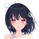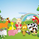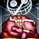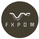1391 lượt xem
Lots of moving averages are based on a weighted sum, the most common ones being the simple (arithmetic) and linearly weighted moving average . The problems with the weighted sum approach is that when your moving average is a FIR filter then the number of operations increase with higher values of length, and when the weights are based on a complex calculation this number of operations can increase drastically!

For the common technical analyst the calculation time of moving averages can be an insignificant factor, even more when using higher time frames, however its always a good practice to seek better performances. The SMA has already a calculation where the number of operations is independent of its length, as such it can be easy to do the same for the linearly weighted moving average ( WMA ). This post will describe the process toward calculating a simple and efficient WMA which will then be used to provide an efficient calculation of the least squares moving average ( LSMA ).

Carving Impulses Responses

Remember that impulses responses fully describe the properties of moving averages, the impulse response of the WMA is a linearly decreasing function, so we'll try to calculate it without using a weighted sum. We first need to use a cumulative sum, the cumulative sum can be described as a summation from the first element of a series to the nth element of the series, where n is the current bar number, one could say that this operation is actually super inefficient, however this is not the case, as a cumulative sum can be calculated recursively as follows:

`y = y + x`

The cumulative sum can be described as an amplifier and posses the following impulse response:

Once the cumulative sum receive the impulse signal as input the result will always be equal to 1. This will form the basis of our simplified calculation, all we need to do transform this response into a linearly decreasing one. The full process is as follows:

• Get the impulse response of the cumulative sum
• Subtract this response from a linearly increasing impulse response of size length
• Normalize the result such that the sum of the resulting response is equal to 1

We need a linearly increasing response of size length, this can be done by using a running sum of the original cumulative sum response, however we must make sure that the value of this response is 0 when the one of the cumulative sum is first equal to 1. Because the resulting response as a maximum value of length we need to multiply our cumulative sum response with length, then we proceed to subtraction.

Finally we need to normalize the result, the sum of a linear sequence of values starting at 1 and ending at n is given by the explicit formula : n(n+1)/2, which in our case give length*(length+1)/2, we divide our previous response with this result and we end up with the impulse response of a WMA . This process can be graphically described as follows:

We can then replace the impulse function by the closing price in order to get the WMA of the closing price.

The big advantage of this calculation is its efficiency, in its non functional form (you can see it in the code) the calculation of the WMA only require 9 operations regardless of the value of length against length*2 + 4 for the weighted sum approach, as such both methods are equally efficient in terms of operations as long as the length of a standard WMA is inferior to 3, which is ridiculous, as such our approach is more appropriate.

Another advantage is that Pinescript does not allow for series as length arguments in the WMA function, however here we can have a variable length for the WMA .

Of course there are disadvantages to this approach, in terms of code we require more variables for the non functional form, which create a lengthier scripts. Another disadvantage is that we can be prone to rounding errors due to the cumulative sum, however they shouldn't be significants in our case.

Getting The Least Squares Moving Average

The LSMA is one of my favorite moving averages, and it can derived from a linear combination between the WMA and SMA described as follows : 3WMA - 2SMA. Since we proposed an alternative calculation of the WMA we can then calculate the LSMA without even using the SMA , why ? because the SMA can be calculated by computing the changes over length period of the cumulative sum of an input, this result is then divided by length.

Remember that the impulse response of a cumulative sum is just a rectangular function, all we need is to truncate it such that only length values of the response are equal to 1, this is done thanks to the change function in Pine.

In Summary

A more efficient calculations for both the WMA and LSMA have been presented, while this on itself isn't super important you have learned what is the process toward calculating a filter without relying on a weighted sum.

This calculation will soon be included in the Pinecoders script allowing series as length argument.

« Je suis las des cruautés de mes semblables, qui ne sont pas mes pareils.

« Je prendrai l’essor et je m’envolerai vers la mer.

« Je connaîtrai le goût des brises du large. J’entendrai les grands cris de la tempête.

## Bình luậnThe script using series as length argument has been updated using the proposed wma and lsma calculations. Note that in the second graph, c (the blue line) has been shifted on purpose, I shouldn't have done that.
Phản hồi